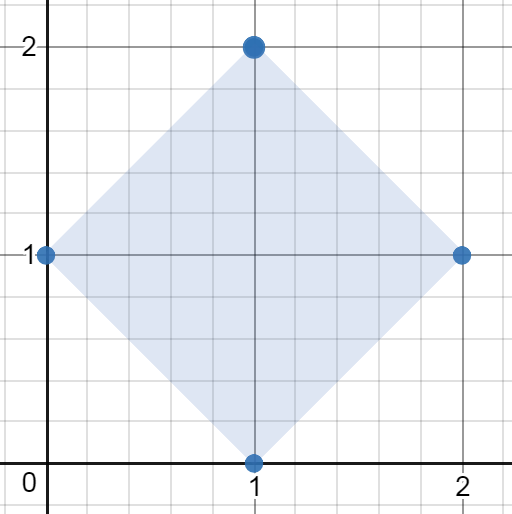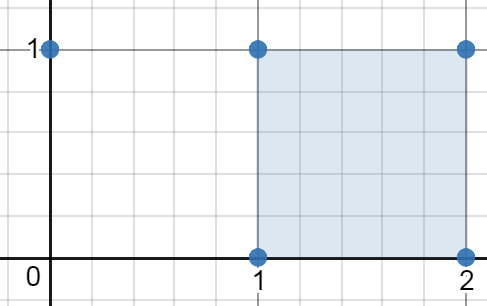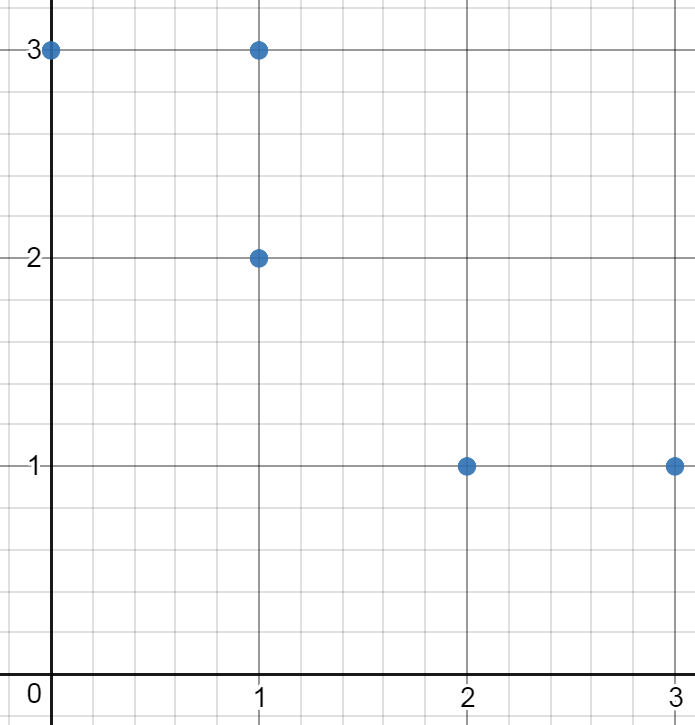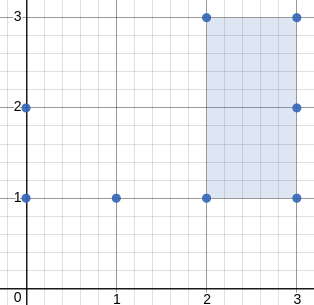# 963. Minimum Area Rectangle II (Medium)

https://leetcode.com/problems/minimum-area-rectangle-ii/

Given a set of points in the xy-plane, determine the minimum area of any rectangle formed from these points, with sides not necessarily parallel to the x and y axes.

If there isn't any rectangle, return 0.

Example 1:```Input: [[1,2],[2,1],[1,0],[0,1]]
Output: 2.00000
Explanation: The minimum area rectangle occurs at [1,2],[2,1],[1,0],[0,1], with an area of 2.
```

Example 2:```Input: [[0,1],[2,1],[1,1],[1,0],[2,0]]
Output: 1.00000
Explanation: The minimum area rectangle occurs at [1,0],[1,1],[2,1],[2,0], with an area of 1.
```

Example 3:```Input: [[0,3],[1,2],[3,1],[1,3],[2,1]]
Output: 0
Explanation: There is no possible rectangle to form from these points.
```

Example 4:```Input: [[3,1],[1,1],[0,1],[2,1],[3,3],[3,2],[0,2],[2,3]]
Output: 2.00000
Explanation: The minimum area rectangle occurs at [2,1],[2,3],[3,3],[3,1], with an area of 2.
```

Note:

1. `1 <= points.length <= 50`
2. `0 <= points[i] <= 40000`
3. `0 <= points[i] <= 40000`
4. All points are distinct.
5. Answers within `10^-5` of the actual value will be accepted as correct.

## Solutions

``````class Solution {
public double minAreaFreeRect(int[][] points) {
int size = points.length;

Point[] oPoints = new Point[size];
Set<Point> pointSet = new HashSet();

for (int i = 0; i < size; ++i) {
oPoints[i] = new Point(points[i], points[i]);
}

double ans = Double.MAX_VALUE;

// it's a brute-force solution as well. the time complexity is O(n^3)
for (int i = 0; i < size; ++i) {
Point p1 = oPoints[i];

for (int j = 0; j < size; ++j) {
if (j == i) {
continue;
}

Point p2 = oPoints[j];

// no need to verify whether the link line of these points are parallel to x or y axis,
// since the problem has already stated that sides not necessarily parallel to the x and y axes.

// start from j + 1, otherwise will bring about redundant computation overhead
for (int k = j + 1; k < size; ++k) {
if (k == i) {
continue;
}

Point p3 = oPoints[k];

// assume p1 is right angle of triangle composed of p1,p2,p3, then vector p1p2 must be
// perpendicular p1p3, which means dot production of p1p2 and p1p3 is 0
int dot = ((p2.x - p1.x) * (p3.x - p1.x) + (p2.y - p1.y) * (p3.y - p1.y));
if (dot != 0) {
continue;
}

// we can use formula p0p4=p0p1 + p1p2 + p2p4 to derive the fourth point, where p0 is the
// original point, the trick here is p2p4 can be substituted by p1p3 since it's a rectangle
// for which the opposite edges are required to be parallel.
Point p4 = new Point(p2.x + p3.x - p1.x, p2.y + p3.y - p1.y);
if (!pointSet.contains(p4)) {
continue;
}

double area = p1.distance(p2) * p1.distance(p3);
if (area < ans) {
ans = area;
}
}
}
}

return ans < Double.MAX_VALUE ? ans : 0;
}

class Point {
// when you implement your own Point class, you have to overwrite the equals and hashCode function, or
// points of same coordinate value will not match
public int x = -1;
public int y = -1;

public Point(int x, int y) {
this.x = x;
this.y = y;
}

@Override
public boolean equals(Object p) {
if (!(p instanceof Point)) {
return false;
}

Point np = (Point)p;

if (np.x != x || np.y != y) {
return false;
}

return true;
}

@Override
public int hashCode() {
return (x + "#" + y).hashCode();
}

public double distance(Point p) {
return Math.sqrt(Math.pow(x - p.x, 2) + Math.pow(y - p.y, 2));
}
}
}
``````